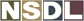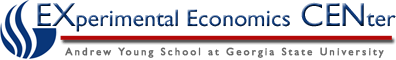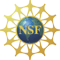Students / Subjects# Duopoly Game

### Market Structure: Duopoly Game

The Cournot model predicts that in a market with a small number of producers, the price will fall between the price in a competitive market and the monopoly price. To illustrate this model, we evaluate a market with two producers.

In Cournot's original analysis, he considered the market for mineral water in a small town, and asked what the price and output would be if there were only two producers who compete with each other. The total output of these two firms is the sum of their individual outputs: Q = q1 + q2 where firm 1 produces q1 and firm 2 produces q2.

This section describes the Cournot duopoly model as a game, and experiment configurations are included for two versions of the game. In a separate section, we evaluate a duopoly market with two sellers and several buyers.

### Cournot duopoly game

One of the two Cournot duopoly games that we have included as an experiment use the market demand, seller costs, and strategy sets that were used for the duopoly experiment reported in Huck, Müller, and Normann . The other duopoly experiment that we have included is a simplified version of the design used by Huck, Müller, and Normann. We start with the simplified version, because its payoff matrix has fewer strategies.

Simple duopoly game experiment

Suppose that there are two firms each with a unit cost c = 0 for each unit produced, and the price is p = 12 - Q, where Q is the sum of the output q1 by firm 1 and q2 by firm 2.

For the price and unit cost above, the profit function for firm 1 is p1(q1, q2) = (12 - q1 - q2) q1; the profit function for firm 2 is similar: p2(q2, q2) = (12 - q1 - q2) q2.

Suppose also that each firm can choose one of 5 output levels, q1 = 2, 3, 4, 5, or 6, and q2 = 2, 3, 4, 5, or 6. The payoffs for both players are shown in the payoff table in figure 1. The row labels represent the possible output choices by firm 1, and the column labels represent the possible output choices by firm 2. The first number in each cell represents the payoff to firm 1, and the second number represents the payoff to firm 2. You can verify that the entries in the payoff matrix are determined from the profit functions above by calculating the values in some cells. For example, in the cell with q1 = 4 and q2 = 3, the price is p = 12 - q1 - q2 = 5, so the profit of firm 1 is p1(4, 3) = 5 × 4 = 20 and the profit function for firm 2 is p2(4, 3) = 5 × 3 = 15.Figure 1: Simple duopoly game payoff table

There are two things that you will want to note about the payoff table in figure 1. First, if both firms produce 4 units (q1 = 4 and q2 = 4), then neither firm can increase its payoff by changing its output, assuming that the other firm doesn't change its output. This is called a Nash equilibrium. Another important point is that this is not the best payoff that can be attained by the two firms. If both firms reduce their output to 3 (so q1 = 3 and q2 = 3) then the profit of each firm would increase from 16 to 18. The total profit that the two firms obtain in the Nash equilibrium, p1 + p2 = 32, is lower than the maximum joint profit that they could earn, which is p1 + p2 = 36, because of the competition between them.

The simple duopoly game above has only five output levels for each choice, so the payoff table is fairly easy to understand. However, there are two aspect of the payoff table that are undesirable. First, not all strategies have a unique best response. Specifically, if Firm 1 chooses 3 (or more accurately, if Firm 2 expects Firm 1 to choose 3) then the best response by Firm 2 is to choose either 4 or 5. Similarly, if Firm 2 expects Firm 1 to choose 5, then the best response by Firm 2 is to choose either 3 or 4. Although this game has a unique pure strategy Nash equilibrium, the formulation of the duopoly game by Huck, Müller, and Normann has a unique best response for each firm to each output choice by the other firm. This proves very useful in the description of a simple dynamic of strategy choices, so we also describe their version of the game, and include a configuration for playing the game.

Original Huck, Müller, and Normann experiment

In the original Huck, Müller, and Normann experiment, each firm had a unit cost of c = 6 for each unit produced, and the price is p = 30 - Q, where Q is the sum of the output q1 by firm 1 and q2 by firm 2.

For the price and unit cost above, the profit function for firm 1 is p1(q1, q2) = (30 - q1 - q2) q1 - 6 q1.

The profit function for firm 2 is similar: p2(q2, q2) = (30 - q1 - q2) q2 - 6 q2.

In the experiment that Huck, Müller, and Normann conducted, they only allowed output levels between 3 and 15 for each player, so we have done the same in the experiment that we prepared. The payoffs for both players are shown in a payoff table. The first number in each cell represents the payoff to firm 1, and the second number represents the payoff to firm 2. You can verify that the entries in the payoff matrix are determined from the profit functions above by calculating the values in some cells. For example, in the cell with q1 = 6 and q2 = 4, the price is p = 20, so the profit of firm 1 is p1(6, 4) = 20 × 6 - 6 × 6 = 84 and the profit function for firm 2 is p2(6, 4) = 20 × 4 - 6 × 4 = 56.

As in the case of the simple duopoly game above, the Nash equilibrium outputs (q1 = 8 and q2 = 8) lead to lower profits for each firm that what they could obtain by colluding and reducing outputs to q1 = 6 and q2 = 6. For these lower output levels, the profit of each firm would increase from 64 to 72. The total profit that the two firms obtain in the Nash equilibrium, p1 + p2 = 128, is lower than the maximum joint profit that they could earn, which is p1 + p2 = 144.

### Duopoly Game Experiment Configurations

If you are familiar with how to run experiments on EconPort, you can click on one of the buttons below to add the experiment to your EconPort profile. If you haven't previously run an EconPort experiment, read about EconPort Configuration and Experiment Setup before you install a configuration. To include the simple duopoly game in your EconPort experiment configuration profile, click on the button below.

To include the Huck, Müller, and Normann duopoly game in your EconPort experiment configuration profile, click on the button below.

References

Cournot, Antoine Augustin (1838). "Recherches sur les principes mathématiques de la théorie des richesses," (translated by N.T. Bacon as Researches into the Mathematical Principles of the Theory of Wealth, 1897).

Huck, Steffan, Wieland Müller, and Hans-Theo Normann (2001). "Stackelberg beats Cournot: On collusion and effciency in experimental markets," Economic Journal 111, pp. 749-766.